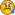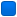###Author Topic: Read INI-File  (Read 5735 times)

#### pitter2206

• Guest« on: March 05, 2011, 07:12:19 pm »
Hi there,

the problem I have is to read an INI-File.
Until now, I worked with wcscmp() like that:
Code: [Select]
` ini  = _wfopen( GPWini, L"r" ); while(fgetws(cText, 2000, ini) != 0) { wcscpy(variable1, cText); if (wcscmp(variable1, L"[GoPal]\n\n") == 0 || wcscmp(variable1, L"[GoPal]\n") == 0 ) iniflag = TRUE; { if (wcscmp(variable1, L"Version=9") == 0 ||  wcscmp(variable1, L"Version=9\n") == 0 ||  wcscmp(variable1, L"Version=9\n\n") == 0 && iniflag == TRUE) { wcscat(variable, L"9xxxx \n"); } } } iniflag = FALSE; fclose(ini);`
But now, I have to read out an individual Versio-Type, which is set by another application... not written in C.

I tried it with GetPrivateProfileString(), but with Windows CE it won´t work.
How can I get this INI-entry?

Greets
Pitter

#### pitter2206

• Guest« Reply #1 on: March 11, 2011, 04:45:46 pm »
No ideas?I tried to read the line and to split it, but it does not work...

####TimoVJL

• Global Moderator
• Member
••• Posts: 1940« Reply #2 on: March 11, 2011, 08:01:06 pm »
This is for finding section [GoPal] and keyname "Version" without comparing versionnumber
Code: [Select]
`#include <wchar.h>#include <stdio.h>int main(int argc, char **argv){ int iniflag; wchar_t cText[2000 + 1], variable; wchar_t GPWini[] = L"GPW.ini"; FILE *ini = _wfopen(GPWini, L"r"); if (ini) { iniflag = 0; while (fgetws(cText, 2000, ini) != 0) { if (cText == L';') continue; // comment if (cText == L'[') // if it is a section if (wcsncmp(cText, L"[GoPal]", 7) == 0) iniflag = 1; else iniflag = 0; if (iniflag > 0 && wcsncmp(cText, L"Version", 7) == 0) { if (cText == L'=' || ((cText == L' ' || cText == L'\t') && cText == L'=')) { wcscat(variable, L"9xxxx \n"); printf("Found: %ls", cText); break; } } } fclose(ini); } return 0;}`GPW.ini
Code: [Select]
`;[GoPal];Version = 8;Version=9;Version = 9Version = 9;[Foo];`
May the source be with you

#### pitter2206

• Guest« Reply #3 on: March 12, 2011, 04:41:32 pm »
Thank you timovjl!

just now, I figured it out like that to get only the characters after the "=":

Code: [Select]
` wchar_t *string = cText;wchar_t *token;wchar_t *status;FILE *ini = _wfopen(GPWini, L"r"); if (ini) { iniflag = 0; while (fgetws(cText, 2000, ini) != 0) { if (cText == L';') continue; // comment if (cText == L'[') // if it is a section if (wcsncmp(cText, L"[GoPal]", 7) == 0) iniflag = 1; else iniflag = 0; if (iniflag > 0 && wcsncmp(cText, L"Version", 7) == 0) { if (cText == L'=' || ((cText == L' ' || cText == L'\t') && cText == L'=')) { token = wcstok(string, L" =", &status); token = wcstok(NULL, L" =", &status); wcscat(variable, token); wcscat(variable, L"\n"); break; } } } fclose(ini); }`

####TimoVJL

• Global Moderator
• Member
••• Posts: 1940« Reply #4 on: March 12, 2011, 09:41:50 pm »
Or this way as offset of '=' is already known
Code: [Select]
`#include <wchar.h>#include <stdio.h>int main(int argc, char **argv){ int iniflag; wchar_t cText[2000 + 1], variable; wchar_t GPWini[] = L"GPW.ini"; FILE *ini = _wfopen(GPWini, L"r"); if (ini) { iniflag = 0; variable = 0; while (fgetws(cText, 2000, ini) != 0) { if (cText == L';') continue; // comment if (cText == L'[') // if it is a section if (wcsncmp(cText, L"[GoPal]", 7) == 0) iniflag = 1; else iniflag = 0; if (iniflag > 0 && wcsncmp(cText, L"Version", 7) == 0) { if (cText == L'=') { wcscat(variable, &cText); break; } if ((cText == L' ' || cText == L'\t') && cText == L'=') { wcscat(variable, &cText); break; } } } printf("Version: %ls", variable); fclose(ini); } return 0;}`
May the source be with you

#### pitter2206

• Guestok...